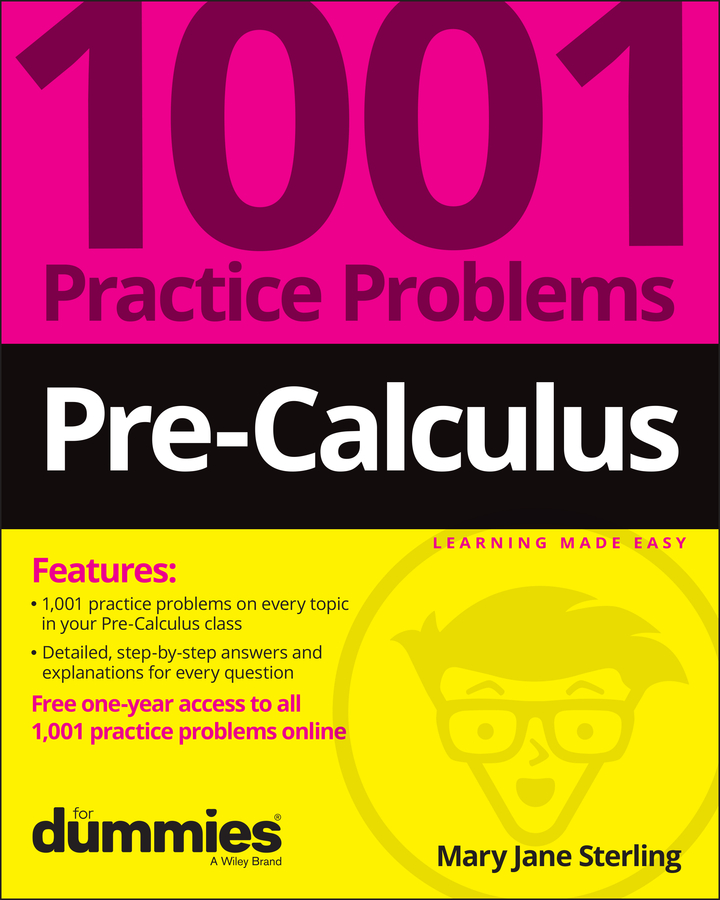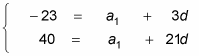##### Pre-Calculus: 1001 Practice Problems For Dummies (+ Free Online Practice)At some point, your pre-calculus teacher will ask you to find the general formula for the nth term of an arithmetic sequence without knowing the first term or the common difference. In this case, you will be given two terms (not necessarily consecutive), and you will use this information to find a1 and d. The steps are: Find the common difference d, write the specific formula for the given sequence, and then find the term you're looking for.

For instance, to find the general formula of an arithmetic sequence where a4 = –23 and a22 = 40, follow these steps:

1. Find the common difference.

You have to be creative in finding the common difference for these types of problems.

1. a.Use the formula an = a1 + (n – 1)d to set up two equations that use the given information.

For the first equation, you know that when n = 4, an = –23:

–23 = a1 + (4 – 1)d

–23 = a1 + 3d

For the second equation, you know that when n = 22, an = 40:

40 = a1 + (22 – 1)d

40 = a1 + 21d

2. b.Set up a system of equations and solve for d.

The system looks like this:You can use elimination or substitution to solve the system. Elimination works nicely because you can multiply either equation by –1 and add the two together to get 63 = 18d. Therefore, d = 3.5.

2. Write the formula for the specific sequence.

This step involves a little work.

1. a.Plug d into one of the equations to solve for a1.

You can plug 3.5 back into either equation:

–23 = a1 + 3(3.5), or a1 = –33.5.

2. b.Use a1 and d to find the general formula for an.

This step becomes a simple three-step simplification:

an = –33.5 + (n – 1)3.5

an = –33.5 + 3.5n – 3.5

an = 3.5n – 37

3. Find the term you were looking for.

In this example, you weren't asked to find any specific term (always read the directions!), but if you were, you could plug that number in for n and then find the term you were looking for.